Euler characteristic

of a finite CW-complexThe integer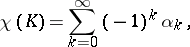whereis the number of-dimensional cells in. It was given this name in honour of L. Euler, who proved in 1758 that the numberof vertices, the numberof edges and the numberof faces of a convex polyhedron are connected by the formula. This relation was known, in an implicit form, already to R. Descartes (1620). It turns out that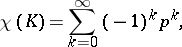where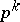is the-dimensional Betti number of the complex(the Euler–Poincaré formula). The Euler characteristic ofis a homology, homotopy and topological invariant of. In particular, it does not depend on the way in which the space is partitioned into cells. Consequently one can speak, for example, of the Euler characteristic of an arbitrary compact polyhedron, meaning by it the Euler characteristic of any of its triangulations. On the other hand, the Euler–Poincaré formula permits the extension of the concept of the Euler characteristic to a larger class of spaces and pairs of spaces for which the right-hand side of the formula remains meaningful. This formula has been generalized to the case of an arbitrary field, where it expresses the Euler characteristic in terms of the dimensions overof the homology groups with coefficients in: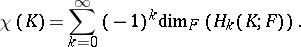Letbe a locally trivial fibration with fibre. If the spaces,andsatisfy certain conditions, then their Euler characteristics are connected by the relation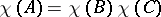. In particular, the Euler characteristic of the direct product of two spaces is equal to the product of their Euler characteristics. The relation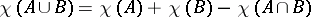, which holds for any excisive triple, makes it possible to compute the Euler characteristics of all compact two-dimensional manifolds. The Euler characteristic of a sphere withhandles anddeleted open discs is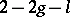, while that of a sphere withMöbius strips anddeleted discs is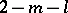. The Euler characteristic of an arbitrary compact orientable manifold of odd dimension is equal to half that of its boundary. In particular, the Euler characteristic of a closed orientable manifold of odd dimension is zero, since its boundary is empty.

Ifis homotopic to the identity mapping of, then the Lefschetz fixed-point theorem (cf. Lefschetz theorem, [a1]) states that if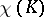is non-zero, thenmust have a fixed point.